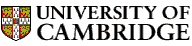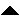Computer LaboratoryComputer Science Syllabus - Foundations of Computer ScienceFoundations of Computer ScienceNext: How to Study Computer Up: Michaelmas Term 2005: Part Previous: Discrete Mathematics I (50%   Contents

## Foundations of Computer Science

Lecturer: Professor L.C. Paulson

No. of lectures and practicals: 15 + 6

Aims

The main aim of this course is to present the basic principles of programming. As the introductory course of the Computer Science Tripos, it caters to students from all backgrounds. To those who have had no programming experience, it will be comprehensible; to those experienced in languages such as C, it will attempt to correct any bad habits that they have learnt.

A further aim is to introduce the principles of data structures and algorithms. The course will emphasise the algorithmic side of programming, focusing on problem-solving rather than on hardware-level bits and bytes. Accordingly it will present basic algorithms for sorting, searching, etc., and discuss their efficiency using O-notation. Worked examples (such as polynomial arithmetic) will demonstrate how algorithmic ideas can be used to build efficient applications.

The course will use a functional language (ML). ML is particularly appropriate for inexperienced programmers, since a faulty program cannot crash. The course will present the elements of functional programming, such as curried and higher-order functions. But it will also discuss traditional (procedural) programming, such as assignments, arrays, pointers and mutable data structures.

Lectures

• Introduction. Levels of abstraction. Floating-point numbers, and why von Neumann was wrong. Why ML? Integer arithmetic. Giving names to values. Declaring functions. Static binding, or declaration versus assignment.

• Recursive functions. Examples: Exponentiation and summing integers. Overloading. Decisions and booleans. Iteration versus recursion.

• O Notation. Examples of growth rates. Dominance. O, Omega and Theta. The costs of some sample functions. Solving recurrence equations.

• Lists. Basic list operations. Append. Naive versus efficient functions for length and reverse. Strings.

• More on lists. The utilities take and drop. Pattern-matching: zip, unzip. A word on polymorphism. The ``making change'' example.

• Sorting. A random number generator. Insertion sort, mergesort, quicksort. Their efficiency.

• Datatypes and trees. Pattern-matching and case expressions. Exceptions. Binary tree traversal (conversion to lists): preorder, inorder, postorder.

• Dictionaries and functional arrays. Functional arrays. Dictionaries: association lists (slow) versus binary search trees. Problems with unbalanced trees.

• Queues and search strategies. Depth-first search and its limitations. Breadth-first search (BFS). Implementing BFS using lists. An efficient representation of queues. Importance of efficient data representation.

• Functions as values. Nameless functions. Currying.

• List functionals. The ``apply to all'' functional, map. Examples: matrix transpose and product. The ``fold'' functionals. Predicate functionals ``filter'' and ``exists''.

• Polynomial arithmetic. Addition, multiplication of polynomials using ideas from sorting, etc.

• Sequences, or lazy lists. Non-strict functions such as IF. Call-by-need versus call-by-name. Lazy lists. Their implementation in ML. Applications, for example Newton-Raphson square roots.

• Elements of procedural programming. Address versus contents. Assignment versus binding. Own variables. Arrays, mutable or not.

Objectives

At the end of the course, students should

• be able to write simple ML programs

• understand the importance of abstraction in computing

• be able to estimate the efficiency of simple algorithms, using the notions of average-case, worse-case and amortised costs

• know the comparative advantages of insertion sort, quick sort and merge sort

• understand binary search and binary search trees

• know how to use currying and higher-order functions

* Paulson, L.C. (1996). ML for the working programmer. Cambridge University Press (2nd ed.).
Okasaki, C. (1998). Purely functional data structures. Cambridge University Press.

Gentler alternative to the main text:
Hansen, M. & Rischel, H. (1999). Introduction to programming using SML. Addison-Wesley.

For reference only:
Gansner, E.R. & Reppy, J.H. (2004). The Standard ML Basis Manual. Cambridge University Press. ISBN: 0521794781Next: How to Study Computer Up: Michaelmas Term 2005: Part Previous: Discrete Mathematics I (50%   Contents
Christine Northeast
Sun Sep 11 15:46:50 BST 2005© 2005 University of Cambridge Computer Laboratory Please send any comments to pagemaster@cl.cam.ac.uk Page last updated on 11-Sep-2005 at 15:57 by Christine Northeast